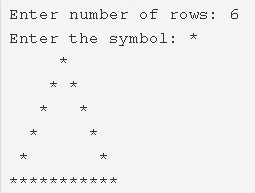# Hollow Pyramid Star Pattern Program in C

C Program to print a hollow pyramid star pattern – In this article, we will explain the several means to print a hollow pyramid star pattern in C programming.

Suitable examples and sample programs have also been added so that you can understand the whole thing very clearly. The compiler has also been added with which you can execute it yourself.

The ways mentioned in this specific piece are as follows:

• Using For Loop
• Using While Loop
• Using Do-While Loop

A pyramid is a three-dimensional figure which has a square as its base and it narrows off into a point giving it the height or the third dimension.

It is a very important figure in the field of geometry. Quite a few monuments all across the world are also famous owing to their shape of a pyramid.

This is what a hollow pyramid looks like:As you can see, you will have to stipulate the number of rows. Afterwards, the pyramid will be printed respectively taking the number of rows into reflection.

Thus, the different methods used to print a hollow pyramid star pattern in C programming are as follows:

## Using For Loop

1. Read the entered number of rows, entered a character and store the values into the variables n, ch.

2) The 1st for loop iterates through rows from i=1 to n with the structure for(i=1;i<=n;i++).

3) The 2nd for loop iterates through columns with the structure for(j=1;j<=n-i;j++) and prints space.

4)For 1st row, nth row

The 3rd for loop iterates through columns with the structure for(j=1;j<=i*2-1;j++).

It prints symbol.

5) For remaining rows other than the 1st row, nth row

The 4th for loop iterates through columns with the structure for(j=1;j<=i*2-1;j++).

Prints symbol if j=1 or j=i*2-1, otherwise it prints space.

6) Cursor comes to next line for each iteration of outer for loop.

Output:

### Using While Loop

1. The while loop is called as an entry checking loop. It checks the condition. If the condition is true then only it executes the code.

2) i=1, The outer while loop iterates through rows until the condition i<=n becomes false.

3) j=1, The 1st Inner while loop iterates through columns until the condition j<=n-i is false.

It prints space.

j value increased by 1.While loop again checks the condition.

4) For i=1 or n

j=1, The 2nd inner while loop iterates through columns until j<=1*2-1 becomes false.

It prints symbol.j value increased by 1.Again checks the condition.

5) For i!=1 or i!=n

j=1, The 3rd inner while loop iterates through columns until the condition j<=1*2-1 becomes false.

if j=1 or j=i*2-1 it prints symbol, otherwise it prints space.

j value increased by 1.Again checks the condition.

6) Cursor comes to next line.i value increased by 1. The outer while loop again checks the condition.

Output:

#### Using Do-While Loop

1. The do-while loop is called as exit checking loop, the loop executes the code once then checks the condition.

i=1, The outer do-while loop iterates through rows.

2) j=1, The 1st inner do-while loop iterates through columns.

It prints space,j value increased by 1.

Checks the condition j<=n-i+1. If the condition is true then the 1st inner loop again iterates. Repeats until the condition becomes false.

3) For i=1 or n

j=1, The 2nd inner do-while loop iterates through columns.

Prints symbol.j value increased by 1.

Then checks the condition j<=i*2-1. If the condition is true then the 2nd inner loop iterates again. Repeats until the condition becomes false.

4) For i!=1 or i!=n

j=1, The 3rd inner do-while loop iterates through columns.

Prints symbol if j=1 or j=i*2-1, otherwise it prints space.

j value increased by 1.

Checks the condition j<=i*2-1. If the condition is true then the 3rd inner loop iterates again. Repeats until the condition becomes false.

5) Cursor comes to next line.

6) i value increased by 1. Then checks the condition i<=n of the outer do-while loop. If the condition is true then the loop iterates again.Repeats until the condition becomes false.

Output:
x

## C Program To Check If Alphabet, Digit or Special Character | C Programs

C program to check for an alphabet, digit or special character – In this article, ...